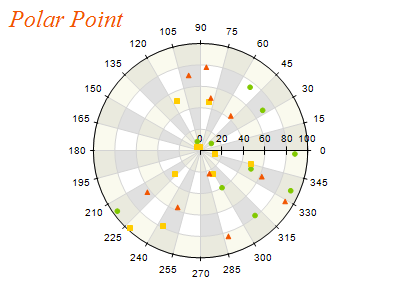﻿ Polar Point
Nevron .NET Vision Documentation
Polar Point

A Polar Point chart displays data points in a 2-dimensional polar coordinate system. The position of a point is specified by its distance from the center and its rotation angle. The following figure displays a Polar Point chart.Figure 1.

Creating a Polar Point series

Polar Point series are represented by the NPolarPointSeries type. An instance of this type must be added to the series collection of a Polar Chart (NPolarChart object).

C#
Copy Code
```// clear the Charts collection and create a new polar chart
NPolarChart chart = new NPolarChart();
chartControl.Charts.Clear();

// add a polar point series to the chart
NPolarPointSeries polarPoint = new NPolarPointSeries();
```
Visual Basic
Copy Code
```' clear the Charts collection and create a new polar chart
Dim chart As New NPolarChart
chartControl.Charts.Clear()

' add a polar point series to the chart
Dim polarPoint As New NPolarPointSeries
```
Passing Data

Once the series is created you can add some data in it. Polar series use the Values data series for the polar data point radiuses (distances from the center). In addition to this data series the polar series provides another data series of type Double, which holds the polar data point angles. It is accessible through the Angles property of the NPolarSeries class.

You can either manipulate directly the Values and Angles data series, or use the data point interface to add data. The NPolarDataPoint class provides several constructors that can be very helpful when you add data points. Please refer to the Working with Data Points topic for more information.

Controlling the points shape

The shape of the point segments can be controlled from the PointShape property. It accepts values from the PointShape enumeration. For example the following code will display the points as pyramids:

C#
Copy Code
```point.PointShape = PointShape.Pyramid;
```
Visual Basic
Copy Code
```point.PointShape = PointShape.Pyramid
```

By default the PointShape property is set to Bar. The size of the points is controlled through the Size property, which is of type NLength. The point size can be specified as percentage of the chart size:

C#
Copy Code
```point.Size = new NLength(1, NRelativeUnit.ParentPercentage);
```
Visual Basic
Copy Code
```point.Size = New NLength(1, NRelativeUnit.ParentPercentage)
```
Formatting commands
Besides the standard formatting commands you can use the following commands in format strings:

<angle_in_degrees> - the current data point angle in degrees (extracted from the Angles data series)
<angle_in_radians> - the current data point angle in radians (extracted from the Angles data series)
Related Examples
Windows forms: Chart Gallery\Polar\Polar Point